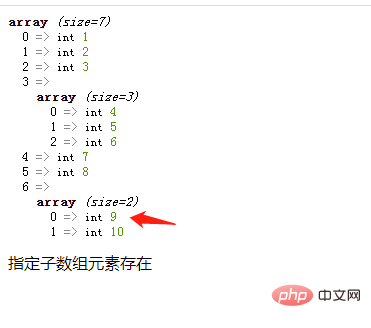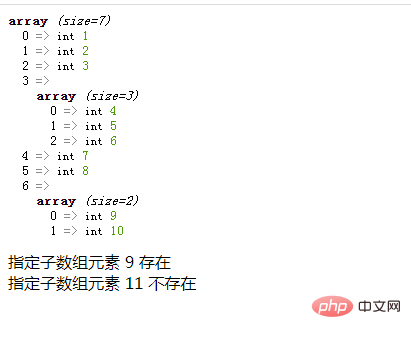# php二维数组中怎么检测子数组元素是否存在php入门到就业线上直播课：进入学习

```foreach(\$arr as \$k => \$v){
//循环体代码
}```
• 遍历给定的 \$arr 数组，在每次循环中会将当前数组的值赋给 \$v，键名赋给 \$k。

• 使用is_array()函数判断外层元素是否为数组类型

• 如果是则使用in_array()函数判断指定元素是否在该子数组中

```if(is_array(\$v)){
if(in_array(指定元素,\$v)){
echo "指定子数组元素存在";
break;
}
}```

```<?php
\$arr = array(1,2,3,array(4,5,6),7,8,array(9,10));
var_dump(\$arr);
foreach(\$arr as \$k => \$v){
if(is_array(\$v)){
if(in_array("9",\$v)){
echo "指定子数组元素存在";
break;
}

}
}
?>``````<?php
function f(\$arr,\$s){
\$c=0;
foreach(\$arr as \$k => \$v){
if(is_array(\$v)){
if(in_array(\$s,\$v)){
\$c=1;
break;
}
}
}
if(\$c==1){
echo "指定子数组元素 \$s 存在<br>";
}else{
echo "指定子数组元素 \$s 不存在<br>";
}
}

\$arr = array(1,2,3,array(4,5,6),7,8,array(9,10));
var_dump(\$arr);
f(\$arr,9);
f(\$arr,11);
?>```in_array() 函数搜索数组中是否存在指定的值。语法格式：

`in_array ( \$search , \$array ,\$strict)`

search必需。规定要在数组搜索的值。
array必需。规定要搜索的数组。
strict 可选。如果该参数设置为 TRUE，则 in_array() 函数检查搜索的数据与数组的值的类型是否相同。1/1